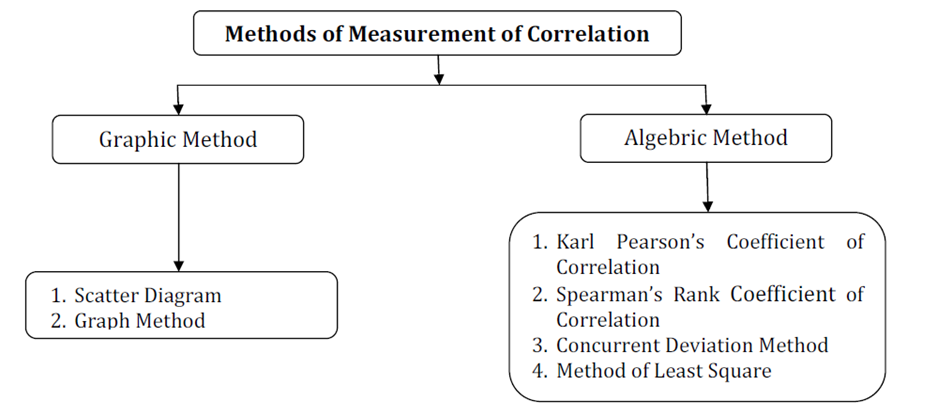# Methods of measurement of correlation

Methods of measurement of correlation:

Quantification of the relationship between variables is very essential to take the benefit of study of correlation. For this, we find there are various methods of measurement of correlation, which can be represented as given below:Among these methods we will discuss only the following methods:

1. Scatter Diagram
2. Karl Pearson’s Coefficient of Correlation
3. Spearman’s Rank Coefficient of Correlation

Scatter Diagram:

This is graphic method of measurement of correlation. It is a diagrammatic representation of bivariate data to ascertain the relationship between two variables. Under this method the given data are plotted on a graph paper in the form of dot. i.e. for each pair of X and Y values we put dots and thus obtain as many points as the number of observations. Usually an independent variable is shown on the X-axis whereas the dependent variable is shown on the Y-axis. Once the values are plotted on the graph it reveals the type of the correlation between variable X and Y. A scatter diagram reveals whether the movements in one series are associated with those in the other series.

Perfect Positive Correlation: In this case, the points will form on a straight line falling from the lower left hand corner to the upper right hand corner

Perfect Negative Correlation: In this case, the points will form on a straight line rising from the upper left hand corner to the lower right hand corner.

High Degree of Positive Correlation: In this case, the plotted points fall in a narrow band, wherein points show a rising tendency from the lower left hand corner to the upper right hand corner.

High Degree of Negative Correlation: In this case, the plotted points fall in a narrow band, wherein points show a declining tendency from upper left hand corner to the lower right hand corner

Low Degree of Positive Correlation: If the points are widely scattered over the diagrams, wherein points are rising from the left hand corner to the upper right hand corner.

Low Degree of Negative Correlation: If the points are widely scattered over the diagrams, wherein points are declining from the upper left hand corner to the lower right hand corner.

Zero (No) Correlation: When plotted points are scattered over the graph haphazardly, then it indicate that there is no correlation or zero.

correlation between two variables.